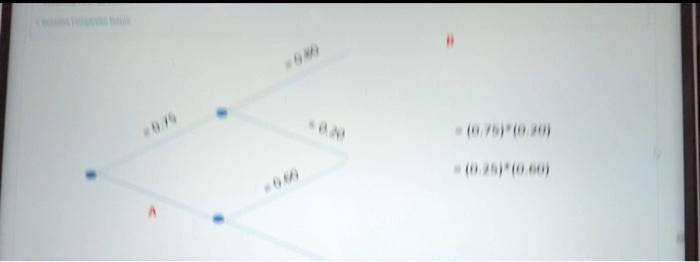Rating : ⭐⭐⭐⭐⭐
Price : \$10.99
Language:EN
Pages: 4
Words: 394

# Calculate Probabilities A, B, C Assignment Answers Needed

75 yards in northern Virginia have a sprinkler system. 80% of the yards with sprinklers are fertilized where 60% of yards without sprinklers are also fertilized.

1. In the space provide the missing values of A,B, and C ?# Step By Step Answers with Explanation

- 75 yards in northern Virginia have a sprinkler system.

To answer this question, we need to define some terms:

- A: The probability that a yard has a sprinkler.

- 60% of yards without sprinklers are fertilized, which means the probability of a yard not having a sprinkler and being fertilized is 0.60. Since A represents the probability of having a sprinkler and not having a sprinkler (complement), we can express this as A(1 - B) = 0.60.

Now, let's solve for A and B:

Now, we need to express C in terms of A and B, since C represents the probability of having a sprinkler and being fertilized:

C = A * B

A * B = 0.80

Now, we can isolate A from this equation:

0.80(1 - B) = 0.60B

0.80 - 0.80B = 0.60B

A = 0.80 / B

A ≈ 0.80 / 0.5714

C = 0.80

2. What is the volume that a randomly selected yard is fertilized?

How It Works